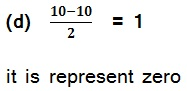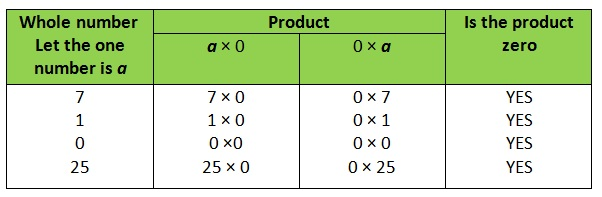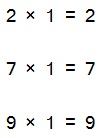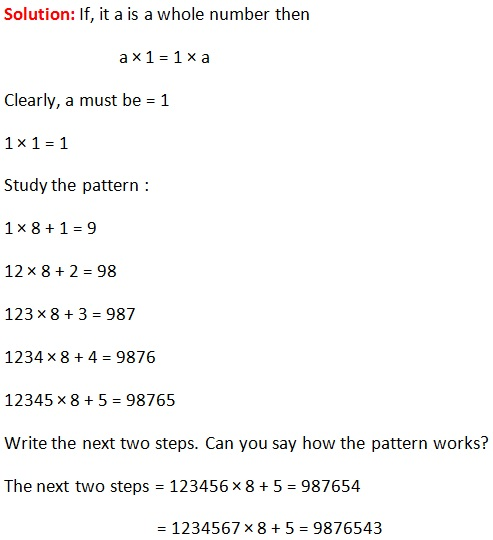Study Materials

# NCERT Solutions for Class 6th Mathematics

Page 3 of 3

## Chapter 2. Whole Numbers

### Exercise-2.3

Q1. Which of the following will not represent zero:
(a) 1 + 0 (b) 0 × 0 (c) 0/2     (d) 10 -10/

Solution:

(a) 1 + 0  = 1

So, it is not represent zero

(b) 0 × 0 = 0

it is represent zero

(c)  0/2 = 0

it is represent zeroQ2. If the product of two whole numbers is zero, can we say that one or both of them will be zero? Justify through examples.

Solution:

Yes, we know that the product of any whole numbers with zero is always zero.Q3. If the product of two whole numbers is 1, can we say that one or both of them will be 1? Justify through examples.

Solution:

If only one number be 1 then the product cannot be 1.

Examples:Here we see that one of two is 1 then product will be always second number.

If both numbers are 1, then the product is 1

Q4. Find using distributive property :
(a) 728 × 101    (b) 5437 × 1001    (c) 824 × 25    (d) 4275 × 125 (e) 504 × 35

Solution:

(a) 728 × 101

= 728(100 + 1)

= 728 × 100 + 728 × 1

= 72800 + 728

= 73528

Solution:

(b) 5437 × 1001

= 5437(1000 + 1)

= 5437 × 1000 + 5437 × 1

= 5437000 + 5437

= 5442437

Solution:

(c) 824 × 25

= 824(20 + 5)

= 824 × 20 + 824 × 5

= 16480 + 4120

20600

Solution:

(d) 4275 × 125

= 4275(100 + 20 + 5)

= 4275 × 100 + 4275 × 20 + 4275 × 5

= 427500 + 85500 + 21375

= 534375

Solution:

(e) 504 × 35

= (500 + 4) × 35

= 500 × 35 + 4 × 35

= 17500 + 140

= 17640

Q5. Study the pattern :
1 × 8 + 1 = 9       1234 × 8 + 4 = 9876
12 × 8 + 2 = 98     12345 × 8 + 5 = 98765
123 × 8 + 3 = 987
Write the next two steps. Can you say how the pattern works?
(Hint: 12345 = 11111 + 1111 + 111 + 11 + 1).Page 3 of 3

Chapter Contents: